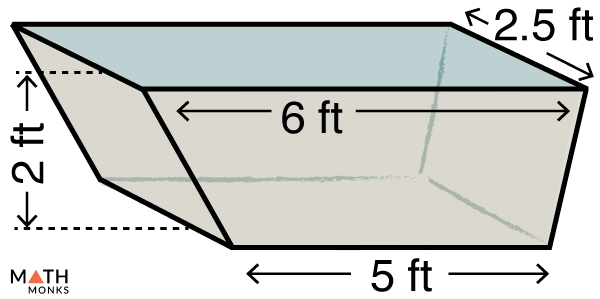# Trapezoidal Prism

A trapezoidal prism is a three-dimensional solid consisting of two identical trapezoidal faces joined together by four rectangular faces. It has 6 faces (2 trapezoidal and 4 rectangular), 12 edges, and 8 vertices..

Some real-life examples of trapezoidal prisms are bathtubs shaped like hollow trapezoidal prisms and blocks or bricks in round structures like fire pits or wells.

A 2-dimensional net can be used to construct a trapezoidal prism to understand its shape. Generally a net is made from paper and cut in a manner such that it can be folded and modified into 3-D shape as shown below.

## Formulas

### Surface Area

The formula is given below:

Total Surface Area (TSA) = (a + b) × h + (a + b + c + d) × l, here a = long base edge, b = short base edge, c and d = slant base edges, h = height, l = length

Alsosince Lateral Surface Area (LSA) = Base perimeter x height

Here, Base Perimeter = (a + b + c + d) × l

Lateral Surface Area (LSA) = (a + b + c + d) × l

Thus, we can write

Total Surface Area (TSA) = (a + b) × h + LSA

Let us solve an example to understand the concept better.

Find the lateral and total surface area of a trapezoidal prism whose base edges are 14 m and 11 m, and slant base edges are 6 m and 8 m. The height is 5 m, and its length is 7 m.

Solution:

As we know,
∴ Lateral Surface Area (LSA) = (a + b + c + d) × l, here a = 14 m, b = 11 m, c = 6 m, d = 8 m, h = 5 m, l = 7 m
LSA = (14 + 11 + 6 + 8) × 7
= 273 m2
Total Surface Area (TSA) = (a + b) × h + LSA, here a = 14 m, b = 11 m, LSA = 273 m2
TSA = (14 + 11) × 5 + 273
= 398 m2

### Volume

The formula is given below:

${Volume\left( V\right) =\dfrac{1}{2}\left( a+b\right) \times h\times l}$, here a = long base edge, b = short base edge, h = height of trapezoidal base, l = length

Let us solve an example to understand the concept better.Find the volume of a trapezoidal prism-shaped bathtub in the figure, and also the volume of water it can hold. (given 1 cubic ft = 28.32 liters)

Solution:

Volume of the trapezoidal prism = Volume of water it can hold
As we know,
${Volume\left( V\right) =\dfrac{1}{2}\left( a+b\right) \times h\times l}$, here a = 6 ft, b = 5 ft, h = 2 ft, l = 2.5 ft,
${\therefore V=\dfrac{1}{2}\times \left( 6+5\right) \times 2×2.5}$
=   27.5 ft3
Converting ft to liters,
Given, 1 cubic ft = 28.32 liters
∴27.5 ft3 = 27.5 × 28.32 liters
= 778.8 liters

• More Resources: